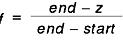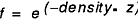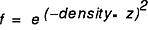[ Previous | Next | Contents | Glossary | Home | Search ]
OpenGL 1.1 for AIX: Reference Manual

## glFog Subroutine

### Purpose

Specifies fog parameters.

### Library

OpenGL C bindings library: libGL.a

### C Syntax

```void glFogf(GLenum ParameterName,
GLfloat ParameterValue)```
```void glFogi(GLenum ParameterName,
GLint ParameterValue)```
```void glFogfv(GLenum ParameterName,
const GLfloat *ParameterValues)```
```void glFogiv(GLenum ParameterName,
const GLint *ParameterValues)```

### Parameters

#### glFogf and glFogi

 ParameterName Specifies a single-valued fog parameter. GL_FOG_DENSITY, GL_FOG_END, GL_FOG_INDEX, GL_FOG_MODE, and GL_FOG_START are accepted. ParameterValue Specifies the value to which ParameterName is set.

#### glFogfv and glFogiv

 ParameterName Specifies a fog parameter. GL_FOG_COLOR, GL_FOG_DENSITY, GL_FOG_END, GL_FOG_INDEX, GL_FOG_MODE, and GL_FOG_START are accepted. ParameterValues Specifies the value or values to be assigned to ParameterName. GL_FOG_COLOR requires an array of four values. All other parameters accept an array containing only a single value.

### Description

The glFog subroutine is enabled and disabled with glEnable and glDisable using the argument GL_FOG. While enabled, fog affects rasterized geometry, bitmaps, and pixel blocks, but not buffer clear operations.

The glFog subroutine assigns the value or values in ParameterValues to the fog parameter specified by ParameterName. The accepted values for ParameterName are:

 GL_FOG_MODE ParameterValues is a single integer or floating-point value that specifies the equation to be used to compute the fog blend factor, f. Three symbolic constants are accepted: GL_LINEAR, GL_EXP, and GL_EXP2. The equations corresponding to these symbolic constants are defined in the following sections. The default fog mode is GL_EXP. GL_FOG_DENSITY ParameterValues is a single integer or floating-point value that specifies Density, the fog density used in both exponential fog equations. Only nonnegative densities are accepted. The default fog density is 1.0. GL_FOG_START ParameterValues is a single integer or floating-point value that specifies Start, the near distance used in the linear fog equation. The default near distance is 0.0. GL_FOG_END ParameterValues is a single integer or floating-point value that specifies End, the far distance used in the linear fog equation. The default far distance is 1.0. GL_FOG_INDEX ParameterValues is a single integer or floating-point value that specifies if, the fog color index. The default fog index is 0.0. GL_FOG_COLOR ParameterValues contains four integer or floating-point values that specify Cf, the fog color. Integer values are mapped linearly such that the most positive representable value maps to 1.0, and the most negative representable value maps to -1.0. Floating-point values are mapped directly. After conversion, all color components are clamped to the range [0,1]. The default fog color is (0,0,0,0).

Fog blends a fog color with each rasterized pixel fragment's post-texturing color using a blending factor f. Factor f is computed in one of three ways, depending on the fog mode. Let z be the distance in eye coordinates from the origin to the fragment being fogged.

The equation for GL_LINEAR fog is:The equation for GL_EXP fog is:The equation for GL_EXP2 fog is:Regardless of the fog mode, f is clamped to the range [0,1] after it is computed. Then, if the GL is in red, green, blue, alpha (RGBA) color mode, the fragment's color, Cr, is replaced by the following:

`Cr prime = fCr + (1 - f) Cf `

In color index mode, the fragment's color index, ir, is replaced by the following:

`ir prime = ir + (1 - f) if `

### Errors

 GL_INVALID_ENUM ParameterName is not an accepted value. GL_INVALID_OPERATION The glFog subroutine is called between a call to glBegin and the corresponding call to glEnd. GL_INVALID_VALUE ParameterName is GL_FOG_DENSITY and ParameterValues is negative.

### Associated Gets

Associated gets for the glFog subroutine are as follows. (See the glGet subroutine for more information.)

glIsEnabled with argument GL_FOG

glGet with argument GL_FOG_COLOR

glGet with argument GL_FOG_INDEX

glGet with argument GL_FOG_DENSITY

glGet with argument GL_FOG_START

glGet with argument GL_FOG_END

glGet with argument GL_FOG_MODE.

### Files

 /usr/include/GL/gl.h Contains C language constants, variable type definitions, and ANSI function prototypes for OpenGL.

### Related Information

The glBegin or glEnd subroutine, glEnable or glDisable subroutine.

[ Previous | Next | Contents | Glossary | Home | Search ]